How Cheenta works to ensure student success?
Explore the Back-Story

# INMO 2012 | ProblemsThis post contains problems from Indian National Mathematics Olympiad, INMO 2012. Try them and share your solution in the comments.

Problem 1

Let ABCD be a quadrilateral inscribed in a circle. Suppose AB =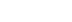and AB subtends 1350 at the center of the circle. Find the maximum possible area of ABCD.

Problem 2

Let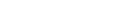and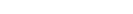be two sets of prime numbers such that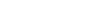and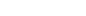. Suppose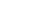and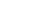. Prove that 30 divides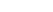.

Problem 3

Define a sequence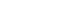n∈N of functions as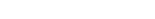,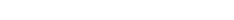, for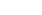. Prove that each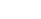is a polynomial with integer coefficients.

Problem 4

Let ABC be a triangle. An interior point P of ABC is said to be good if we can find exactly 27 rays emanating from P intersecting the sides of the triangle ABC such that the triangle is divided by these rays into 27 smaller triangles of equal area. Determine the number of good points for a given triangle ABC.

Problem 5

Let ABC be an acute angled triangle. Let D, E, F be points on BC, CA, AB such that AD is the median, BE is the internal bisector and CF is the altitude. Suppose that angle FDE = angle C and angle DEF = angle A and angle EFD = angle B. Show that ABC is equilateral.

Problem 6

Let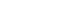be a function satisfying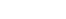,and

1.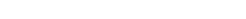,
2.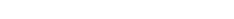for all x ,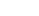simultaneously.
1. Find the set of all possible values of the function f.
2. If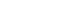and, find the set of all integers n such that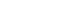.

RMO 1990 Problems

INMO 2018 Problem 6 - Video

This post contains problems from Indian National Mathematics Olympiad, INMO 2012. Try them and share your solution in the comments.

Problem 1

Let ABCD be a quadrilateral inscribed in a circle. Suppose AB =and AB subtends 1350 at the center of the circle. Find the maximum possible area of ABCD.

Problem 2

Letandbe two sets of prime numbers such thatand. Supposeand. Prove that 30 divides.

Problem 3

Define a sequencen∈N of functions as,, for. Prove that eachis a polynomial with integer coefficients.

Problem 4

Let ABC be a triangle. An interior point P of ABC is said to be good if we can find exactly 27 rays emanating from P intersecting the sides of the triangle ABC such that the triangle is divided by these rays into 27 smaller triangles of equal area. Determine the number of good points for a given triangle ABC.

Problem 5

Let ABC be an acute angled triangle. Let D, E, F be points on BC, CA, AB such that AD is the median, BE is the internal bisector and CF is the altitude. Suppose that angle FDE = angle C and angle DEF = angle A and angle EFD = angle B. Show that ABC is equilateral.

Problem 6

Letbe a function satisfying,and

1.,
2.for all x ,simultaneously.
1. Find the set of all possible values of the function f.
2. Ifand, find the set of all integers n such that.

RMO 1990 Problems

INMO 2018 Problem 6 - Video

This site uses Akismet to reduce spam. Learn how your comment data is processed.

### Knowledge Partner